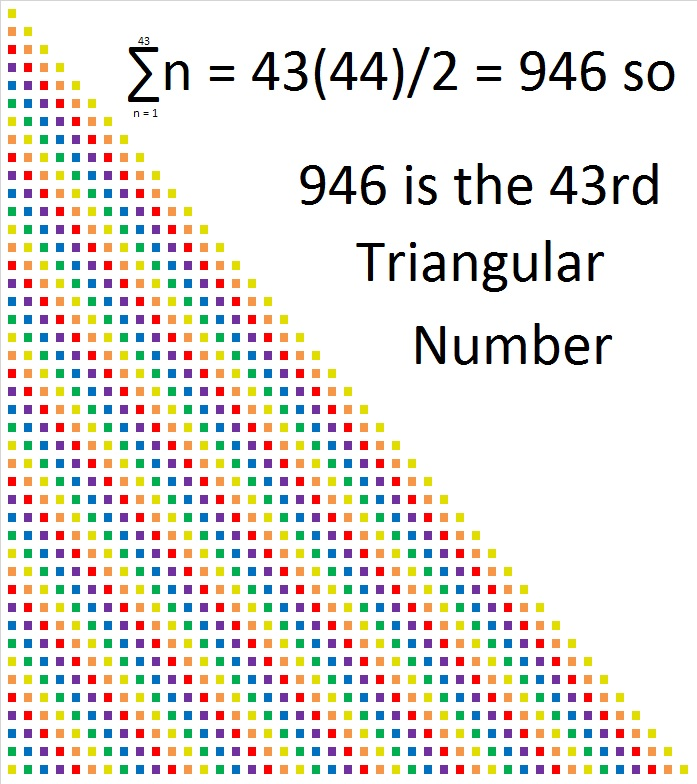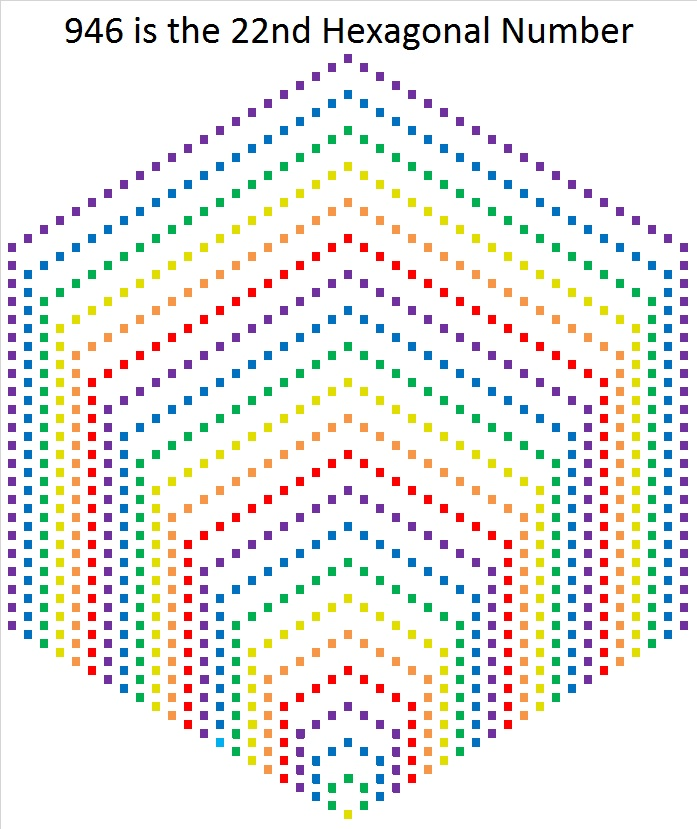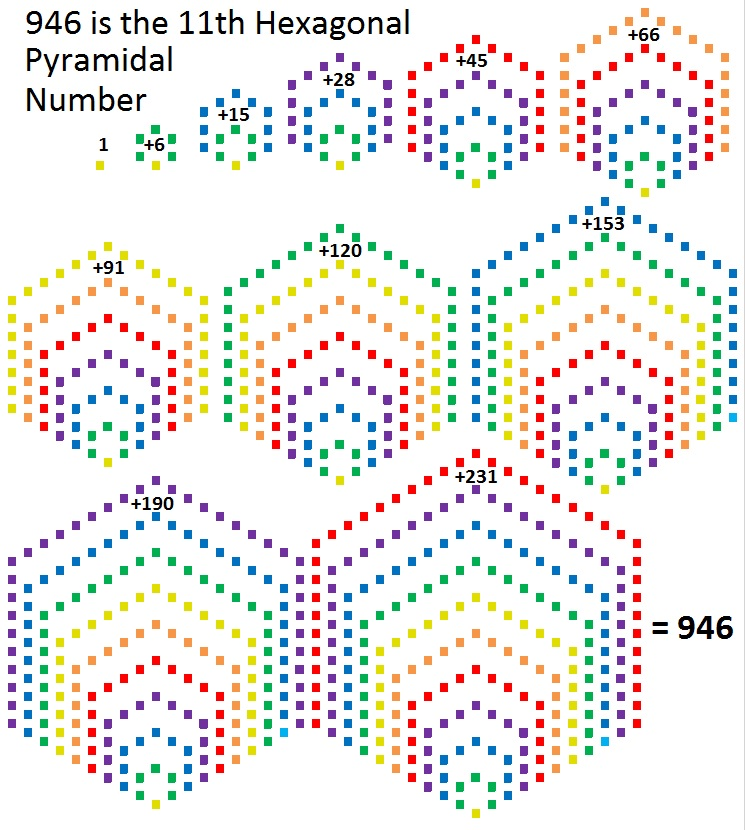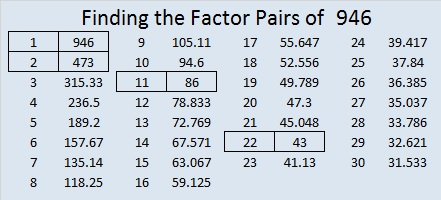# What Kind of Shape Is 946 In?

First of all, 946 is the sum of the numbers from 1 to 43, so it is the 43rd triangular number.Every other triangular number is also a hexagonal number. Since 946 is the 43rd triangular number, and 43 is an odd number, 946 is also the 22nd hexagonal number. 946 is the 22nd hexagonal number because 22(2(22) – 1) = 22(43) = 946.But that’s not all. 946 is different than any previous hexagonal number. 946 is the smallest hexagonal number that is also a hexagonal pyramidal number. It is, in fact, the 11th hexagonal pyramidal number. That means if you stack the hexagons in the graphic below in order from largest to smallest, you would get a hexagonal pyramid made with 946 tiny squares. That’s pretty cool, I think.467 + 479 = 946 so 946 is the sum of two consecutive prime numbers.
946 is also the sum of the twenty prime numbers from 11 to 89.

946 is palindrome 181 in BASE 27 because
1(27²) + 8(27¹) + 1(27⁰) = 729 + 216 + 1 = 946

• 946 is a composite number.
• Prime factorization: 946 = 2 × 11 × 43
• The exponents in the prime factorization are 1, 1, and 1. Adding one to each and multiplying we get (1 + 1)(1 + 1)(1 + 1) = 2 × 2 × 2 = 8. Therefore 946 has exactly 8 factors.
• Factors of 946: 1, 2, 11, 22, 43, 86, 473, 946
• Factor pairs: 946 = 1 × 946, 2 × 473, 11 × 86, or 22 × 43
• 946 has no square factors that allow its square root to be simplified. √946 ≈ 30.75711This site uses Akismet to reduce spam. Learn how your comment data is processed.